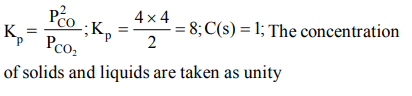## Chemical Equilibrium Questions and Answers Part-11

1. A vessel at 1000 K contains $CO_{2}$ with a pressure of 0.5 atm. Some of the $CO_{2}$ is converted into CO on the addition of graphite. If the total pressure at equilibrium is 0.8 atm, the value of K is :
a) 1.8 atm
b) 3 atm
c) 0.3 atm
d) 0.18 atm

Explanation: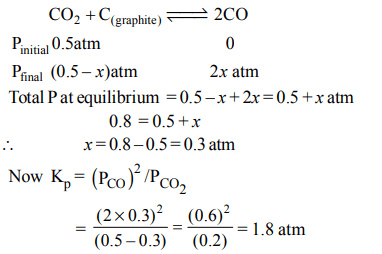2. The equilibrium constant $\left(K_{c}\right)$ for the reaction $N_{2}\left(g\right)+ O_{2}\left(g\right)\rightarrow 2NO\left(g\right)$     at temperature T is $4 × 10^{-4}$  . The value of $\left(K_{c}\right)$ for the reaction $NO\left(g\right)\rightarrow \frac{1}{2}N_{2}\left(g\right)+\frac{1}{2} O_{2}\left(g\right)$     at the same temperature is
a) 0.02
b) $2.5 × 10^{2}$
c) $4 × 10^{-4}$
d) 50.0

Explanation: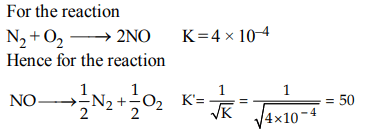3. The decomposition of $N_{2}O_{4}$ to $NO_{2}$   is carried out at 280 K in chloroform. When equilibrium has been established, 0.2 mol of $N_{2}O_{4}$  and $2\times10^{-3}$ mol of $NO_{2}$  are present in a 2l solution. The equilibrium constant for the reaction, $N_{2}O_{4} \rightleftharpoons 2NO_{2}$    is
a) $1 × 10^{-2}$
b) $2 × 10^{-3}$
c) $1 × 10^{-5}$
d) $2 × 10^{-5}$

Explanation: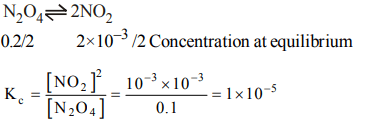4. In an equilibrium reaction for which $\triangle G^{\circ}=0$   , the equilibrium constant, K , should be equal to
a) 0
b) 1
c) 2
d) 10

Explanation: $\triangle$G°= - RT ln Kc for $\triangle$G°= 0 , Kc = 1; since ln 1 = 0

5. The pressure change due to which equilibrium is not affected is
a) $N_{2}\left(g\right)+O_{2}\left(g\right)\rightleftharpoons 2NO\left(g\right)$
b) $2SO_{2}\left(g\right)+O_{2}\left(g\right)\rightleftharpoons 2SO_{3}\left(g\right)$
c) $2O_{3}\left(g\right)\rightleftharpoons 3O_{2}\left(g\right)$
d) $2NO_{2}\left(g\right)\rightleftharpoons N_{2}O_{4}\left(g\right)$

Explanation: When moles of gaseous reactants and the products are the same on both sides, their is no effect of pressure

6. A reversible chemical reaction having two reactants in equilibrium. If the concentration of the reactants are doubled then the equilibrium constant will
a) be doubled
b) be halved
c) become one-fourth
d) remain same

Explanation: The equilibrium constant does not vary with any factor other than temperature

7. 3.2 moles of hydrogen iodide were heated in a sealed bulb at 444°C till the equilibrium state was reached. Its degree of dissociation at this temperature was found to be 22% The number of moles of hydrogen iodide present at equilibrium are
a) 2.496
b) 1.87
c) 2
d) 4

Explanation: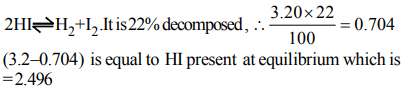8. For the reaction $H_{2}\left(g\right)+I_{2}\left(g\right)\rightleftharpoons 2HI\left(g\right)$     at 721 K, the value of equilibrium constant is 50, when equilibrium concentration of both is 5M. Value of Kp under the same conditions will be
a) 0.02
b) 0.2
c) 50
d) 50 RT

Explanation:9. For which one of the following systems at equilibrium, at constant temperature will the doubling of the volume cause a shift to the right?
a) $H_{2}\left(g\right)+Cl_{2}\left(g\right)\rightleftharpoons 2HCl\left(g\right)$
b) $2CO\left(g\right)+ O_{2}\left(g\right)\rightleftharpoons 2CO_{2}\left(g\right)$
c) $N_{2}\left(g\right)+3H_{2}\left(g\right)\rightleftharpoons 2NH_{3}\left(g\right)$
d) $PCl_{5}\left(g\right)\rightleftharpoons PCl_{3}\left(g\right)+Cl_{2}\left(g\right)$

10. For the reaction $C\left(s\right)+CO_{2}\left(g\right)\rightleftharpoons 2CO\left(g\right)$     , the partial pressures of $CO_{2}$ and CO are 2.0 and 4.0 atm respectively at equilibrium. The $K_{P}$ for the reaction is